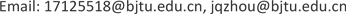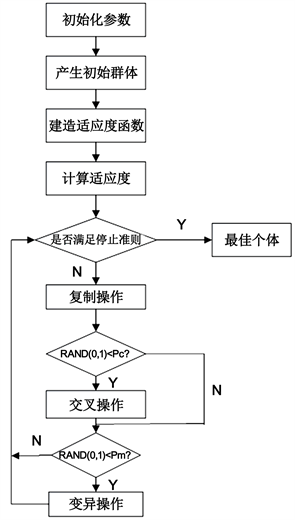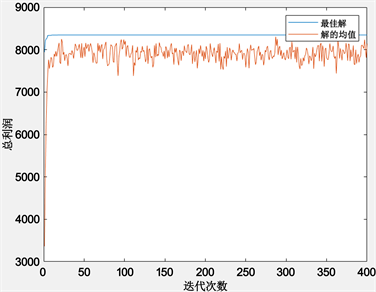﻿ 基于遗传算法的生鲜超市水果库存控制研究 Research on Inventory Control of Fresh Supermarket Fruit Based on Genetic Algorithm

Management Science and Engineering
Vol. 08  No. 02 ( 2019 ), Article ID: 29586 , 11 pages
10.12677/MSE.2019.82015

Research on Inventory Control of Fresh Supermarket Fruit Based on Genetic Algorithm

Ai Zhang, Jianqin Zhou

School of Economics and Management, Beijing Jiaotong University, BeijingReceived: Mar. 13th, 2019; accepted: Mar. 28th, 2019; published: Apr. 4th, 2019ABSTRACT

With the gradual improvement of people’s consumption level, people’s demand for healthy, nutritious and safe fruits and vegetables has become increasingly high. At the same time, they turned to be too picky on the freshness of the fruits and vegetables, which makes it harder for the administrator to operate the fruit with seasonal, perishability and short shelf life. The operating profit of fresh supermarket is largely determined by the inventory control level, and the scientific and effective inventory control strategy is an effective way to improve the inventory management level of fresh supermarket. This paper constructs an inventory control model considering the characteristics of fresh supermarket business hours and consumer demand affected by price and freshness. Then combined with the S fresh supermarket American cherry sales data as an example, the genetic algorithm of binary coding is used to solve the model. The sale profit increased 13.7% which achieved the rationality of fruit inventory control.

Keywords:Fresh Fruit, Inventory Control, Genetic AlgorithmCopyright © 2019 by author(s) and Hans Publishers Inc.1. 引言

2. 易腐品库存控制研究现状

3. 生鲜超市水果库存控制模型构建

3.1. 问题描述

3.2. 模型假设

1) 水果的订货周期为n，在第n天通过调价或者扔掉使得当日结束时库存为0。

2) 假设不允许缺货，即当库存量将为0时，生鲜水果采销负责人在该天销售结束时立即发出订单通知，发出订货请求后供应商凌晨送货到门店。

3) 水果在超市进行销售时变质速率为常数θ。

4) 一个订货周期内的各项采购成本、单次订购的固定成本、单位库存持有成本不发生变化且为常数。

3.3. 参数说明

1) 决策变量

n：订货周期；

pw：第w个销售日的销售价格；

2) 主要参数

L：水果的销售时间长度；

n：订货周期；

m：水果的货架期；

Q：订购批量；

t：一个销售日的销售时刻；

t1：超市开始营业时间点；

θ：水果的变质率；

β：水果最初到达超市的新鲜度；

w：第w个销售日；

qw:第w个销售日的初始库存量；

Iw(t)：第w个销售日t时刻的库存量；

Dw(t):第w个销售日t时刻的需求量；

Hw(n)：第w个销售日的库存持有成本；

H(n)：总库存持有成本；

Rw(n)：第w个销售日的销售收入；

R(n)：总销售收入；

$\text{π}\left(n,{p}_{1},{p}_{2},{p}_{3},\cdots ,{p}_{n}\right)$ ：总销售利润；

p0：水果的平均市场销售价格；

Cb：单位水果的采购价格；

Ch：单位水果的库存持有成本；

Cd：每次订货的固定成本；

Cr:线下渠道运营成本；

Co：线上渠道运营成本；

η：客流量限制因子。

3.4. 模型构建

$\frac{\text{d}I\left(t\right)}{\text{d}t}=-\theta \left(t\right)I\left(t\right)-D\left(t\right)$ (1)

${D}_{w}\left(t\right)=\left\{\begin{array}{l}\left(a-b{p}_{w}\right){\beta }^{\left(w-1\right)+t}\text{\hspace{0.17em}}\text{\hspace{0.17em}}\text{\hspace{0.17em}}{t}_{1}\le t\le 1\\ 0\text{\hspace{0.17em}}\text{\hspace{0.17em}}\text{\hspace{0.17em}}\text{\hspace{0.17em}}\text{\hspace{0.17em}}\text{\hspace{0.17em}}\text{\hspace{0.17em}}\text{\hspace{0.17em}}\text{\hspace{0.17em}}\text{\hspace{0.17em}}\text{\hspace{0.17em}}\text{\hspace{0.17em}}\text{\hspace{0.17em}}\text{\hspace{0.17em}}\text{\hspace{0.17em}}\text{\hspace{0.17em}}\text{\hspace{0.17em}}\text{\hspace{0.17em}}\text{\hspace{0.17em}}\text{\hspace{0.17em}}\text{\hspace{0.17em}}\text{\hspace{0.17em}}\text{\hspace{0.17em}}\text{\hspace{0.17em}}0\le t\le {t}_{1}\end{array}$ (2)

$\frac{\text{d}I\left(t\right)}{\text{d}t}=\left\{\begin{array}{l}-\theta {I}_{w}\left(t\right)-\left(a-b{p}_{w}\right){\beta }^{\left(w-1\right)+t}\text{\hspace{0.17em}}\text{\hspace{0.17em}}\text{\hspace{0.17em}}{t}_{1}\le t\le 1\\ -\theta {I}_{w}\left(t\right)\text{\hspace{0.17em}}\text{\hspace{0.17em}}\text{\hspace{0.17em}}\text{\hspace{0.17em}}\text{\hspace{0.17em}}\text{\hspace{0.17em}}\text{\hspace{0.17em}}\text{\hspace{0.17em}}\text{\hspace{0.17em}}\text{\hspace{0.17em}}\text{\hspace{0.17em}}\text{\hspace{0.17em}}\text{\hspace{0.17em}}\text{\hspace{0.17em}}\text{\hspace{0.17em}}\text{\hspace{0.17em}}\text{\hspace{0.17em}}\text{\hspace{0.17em}}\text{\hspace{0.17em}}\text{\hspace{0.17em}}\text{\hspace{0.17em}}\text{\hspace{0.17em}}\text{\hspace{0.17em}}\text{\hspace{0.17em}}\text{\hspace{0.17em}}\text{\hspace{0.17em}}\text{\hspace{0.17em}}\text{\hspace{0.17em}}\text{\hspace{0.17em}}0\le t\le {t}_{1}\end{array}$ (3)

${I}_{w}\left(t\right)=\left\{\begin{array}{c}\frac{\left(b{p}_{w}-a\right){\beta }^{\left(w-1\right)+t}}{\mathrm{ln}\beta +\theta }+{c}_{1}{\text{e}}^{-\theta t}\text{\hspace{0.17em}}\text{\hspace{0.17em}}\text{\hspace{0.17em}}\text{\hspace{0.17em}}\text{\hspace{0.17em}}\text{\hspace{0.17em}}\text{\hspace{0.17em}}\text{\hspace{0.17em}}\text{\hspace{0.17em}}\text{\hspace{0.17em}}{t}_{1}\le t\le 1\\ {c}_{2}{\text{e}}^{-\theta t}\text{\hspace{0.17em}}\text{\hspace{0.17em}}\text{\hspace{0.17em}}\text{\hspace{0.17em}}\text{\hspace{0.17em}}\text{\hspace{0.17em}}\text{\hspace{0.17em}}\text{\hspace{0.17em}}\text{\hspace{0.17em}}\text{\hspace{0.17em}}\text{\hspace{0.17em}}\text{\hspace{0.17em}}\text{\hspace{0.17em}}\text{\hspace{0.17em}}\text{\hspace{0.17em}}\text{\hspace{0.17em}}\text{\hspace{0.17em}}\text{\hspace{0.17em}}\text{\hspace{0.17em}}\text{\hspace{0.17em}}\text{\hspace{0.17em}}\text{\hspace{0.17em}}\text{\hspace{0.17em}}\text{\hspace{0.17em}}\text{\hspace{0.17em}}\text{\hspace{0.17em}}\text{\hspace{0.17em}}\text{\hspace{0.17em}}\text{\hspace{0.17em}}\text{\hspace{0.17em}}\text{\hspace{0.17em}}\text{\hspace{0.17em}}\text{\hspace{0.17em}}\text{\hspace{0.17em}}\text{\hspace{0.17em}}\text{\hspace{0.17em}}\text{\hspace{0.17em}}\text{\hspace{0.17em}}0\le t\le {t}_{1}\end{array}$ (4)

$\frac{\left(b{p}_{w}-a\right){\beta }^{\left(w-1\right)+{t}_{1}}}{\mathrm{ln}\beta +\theta }+{c}_{1}{\text{e}}^{-\theta {t}_{1}}={q}_{w}{\text{e}}^{-\theta {t}_{1}}$ (5)

$\begin{array}{l}{c}_{1}=\frac{1}{\mathrm{ln}\beta +\theta }\left(a{e}^{\theta {t}_{1}}{\beta }^{\left(w-1\right)+{t}_{1}}-b{p}_{w}{\text{e}}^{\theta {t}_{1}}{\beta }^{\left(w-1\right)+{t}_{1}}+\mathrm{ln}\beta {q}_{w}\theta {q}_{w}\right)\\ \text{\hspace{0.17em}}\text{\hspace{0.17em}}\text{\hspace{0.17em}}=\frac{{\text{e}}^{\theta {t}_{1}}\left(a-b{p}_{w}\right){\beta }^{{}^{\left(w-1\right)+{t}_{1}}}}{\mathrm{ln}\beta +\theta }+{q}_{w}\end{array}$ (6)

${I}_{w}\left(t\right)=\left\{\begin{array}{c}{q}_{w}{\text{e}}^{-\theta t}+\frac{\left(b{p}_{w}-a\right)}{\mathrm{ln}\beta +\theta }{\beta }^{\left(w-1\right)}\left[{\beta }^{t}-{\beta }^{{t}_{1}}{\text{e}}^{-\theta \left(t-{t}_{1}\right)}\right]\text{\hspace{0.17em}}\text{\hspace{0.17em}}\text{\hspace{0.17em}}\text{\hspace{0.17em}}\text{\hspace{0.17em}}\text{\hspace{0.17em}}\text{\hspace{0.17em}}\text{\hspace{0.17em}}\text{\hspace{0.17em}}\text{\hspace{0.17em}}\text{\hspace{0.17em}}{t}_{1}\le t\le 1\\ {q}_{w}{\text{e}}^{-\theta t}\text{\hspace{0.17em}}\text{\hspace{0.17em}}\text{\hspace{0.17em}}\text{\hspace{0.17em}}\text{\hspace{0.17em}}\text{\hspace{0.17em}}\text{\hspace{0.17em}}\text{\hspace{0.17em}}\text{\hspace{0.17em}}\text{\hspace{0.17em}}\text{\hspace{0.17em}}\text{\hspace{0.17em}}\text{\hspace{0.17em}}\text{\hspace{0.17em}}\text{\hspace{0.17em}}\text{\hspace{0.17em}}\text{\hspace{0.17em}}\text{\hspace{0.17em}}\text{\hspace{0.17em}}\text{\hspace{0.17em}}\text{\hspace{0.17em}}\text{\hspace{0.17em}}\text{\hspace{0.17em}}\text{\hspace{0.17em}}\text{\hspace{0.17em}}\text{\hspace{0.17em}}\text{\hspace{0.17em}}\text{\hspace{0.17em}}\text{\hspace{0.17em}}\text{\hspace{0.17em}}\text{\hspace{0.17em}}\text{\hspace{0.17em}}\text{\hspace{0.17em}}\text{\hspace{0.17em}}\text{\hspace{0.17em}}\text{\hspace{0.17em}}\text{\hspace{0.17em}}\text{\hspace{0.17em}}\text{\hspace{0.17em}}\text{\hspace{0.17em}}\text{\hspace{0.17em}}\text{\hspace{0.17em}}\text{\hspace{0.17em}}\text{\hspace{0.17em}}\text{\hspace{0.17em}}\text{\hspace{0.17em}}\text{\hspace{0.17em}}\text{\hspace{0.17em}}\text{\hspace{0.17em}}\text{\hspace{0.17em}}\text{\hspace{0.17em}}\text{\hspace{0.17em}}\text{\hspace{0.17em}}\text{\hspace{0.17em}}\text{\hspace{0.17em}}\text{\hspace{0.17em}}\text{\hspace{0.17em}}0\le t\le {t}_{1}\end{array}$ (7)

${I}_{w}\left(t\right)=\left\{\begin{array}{c}{q}_{w}{\text{e}}^{-\theta t}+\frac{\left(b{p}_{w}-a\right)}{\mathrm{ln}\beta +\theta }\left[{\text{e}}^{\left[\left(w-1\right)+t\right]\mathrm{ln}\beta }-{\text{e}}^{\left(w-1\right)\mathrm{ln}\beta +\left(\mathrm{ln}\beta +\theta \right){t}_{1}-\theta t}\right]\text{\hspace{0.17em}}\text{\hspace{0.17em}}\text{\hspace{0.17em}}\text{\hspace{0.17em}}\text{\hspace{0.17em}}\text{\hspace{0.17em}}\text{\hspace{0.17em}}\text{\hspace{0.17em}}\text{\hspace{0.17em}}\text{\hspace{0.17em}}\text{\hspace{0.17em}}{t}_{1}\le t\le 1\\ {q}_{w}{\text{e}}^{-\theta t}\text{\hspace{0.17em}}\text{\hspace{0.17em}}\text{\hspace{0.17em}}\text{\hspace{0.17em}}\text{\hspace{0.17em}}\text{\hspace{0.17em}}\text{\hspace{0.17em}}\text{\hspace{0.17em}}\text{\hspace{0.17em}}\text{\hspace{0.17em}}\text{\hspace{0.17em}}\text{\hspace{0.17em}}\text{\hspace{0.17em}}\text{\hspace{0.17em}}\text{\hspace{0.17em}}\text{\hspace{0.17em}}\text{\hspace{0.17em}}\text{\hspace{0.17em}}\text{\hspace{0.17em}}\text{\hspace{0.17em}}\text{\hspace{0.17em}}\text{\hspace{0.17em}}\text{\hspace{0.17em}}\text{\hspace{0.17em}}\text{\hspace{0.17em}}\text{\hspace{0.17em}}\text{\hspace{0.17em}}\text{\hspace{0.17em}}\text{\hspace{0.17em}}\text{\hspace{0.17em}}\text{\hspace{0.17em}}\text{\hspace{0.17em}}\text{\hspace{0.17em}}\text{\hspace{0.17em}}\text{\hspace{0.17em}}\text{\hspace{0.17em}}\text{\hspace{0.17em}}\text{\hspace{0.17em}}\text{\hspace{0.17em}}\text{\hspace{0.17em}}\text{\hspace{0.17em}}\text{\hspace{0.17em}}\text{\hspace{0.17em}}\text{\hspace{0.17em}}\text{\hspace{0.17em}}\text{\hspace{0.17em}}\text{\hspace{0.17em}}\text{\hspace{0.17em}}\text{\hspace{0.17em}}\text{\hspace{0.17em}}\text{\hspace{0.17em}}\text{\hspace{0.17em}}\text{\hspace{0.17em}}\text{\hspace{0.17em}}\text{\hspace{0.17em}}\text{\hspace{0.17em}}\text{\hspace{0.17em}}\text{\hspace{0.17em}}\text{\hspace{0.17em}}\text{\hspace{0.17em}}\text{\hspace{0.17em}}\text{\hspace{0.17em}}\text{\hspace{0.17em}}\text{\hspace{0.17em}}\text{\hspace{0.17em}}\text{\hspace{0.17em}}\text{\hspace{0.17em}}\text{\hspace{0.17em}}\text{\hspace{0.17em}}\text{\hspace{0.17em}}0\le t\le {t}_{1}\end{array}$ (8)

$\begin{array}{l}{q}_{w+1}=\frac{{\text{e}}^{-\theta }}{\mathrm{ln}\beta +\theta }\left({q}_{w}\left(\mathrm{ln}\beta +\theta \right)-\left(a-b{p}_{w}\right)\left({\text{e}}^{w\mathrm{ln}\beta +\theta }-{\text{e}}^{\theta {t}_{1}+\mathrm{ln}\beta \left[\left(w-1\right)+{t}_{1}\right]}\right)\right)\\ \text{\hspace{0.17em}}\text{\hspace{0.17em}}\text{\hspace{0.17em}}\text{\hspace{0.17em}}\text{\hspace{0.17em}}\text{\hspace{0.17em}}=\frac{{\text{e}}^{-\theta }}{\mathrm{ln}\beta +\theta }\left({q}_{w}\left(\mathrm{ln}\beta +\theta \right)-\left(a-b{p}_{w}\right){\text{e}}^{w\mathrm{ln}\beta }\left({\text{e}}^{\theta }-{\text{e}}^{\theta {t}_{1}+\mathrm{ln}\beta \left[-1+{t}_{1}\right]}\right)\right)\\ \text{\hspace{0.17em}}\text{\hspace{0.17em}}\text{\hspace{0.17em}}\text{\hspace{0.17em}}\text{\hspace{0.17em}}\text{\hspace{0.17em}}={q}_{w}{\text{e}}^{-\theta }-\frac{\left(1-{\text{e}}^{\left(-\mathrm{ln}\beta \right)\left(1-{t}_{1}\right)-\theta \left(1-{t}_{1}\right)}\right)\left(\left(a-b{p}_{w}\right){\text{e}}^{w\mathrm{ln}\beta }\right)}{in\beta +\theta }\end{array}$ (9)

${q}_{w+1}={q}_{w}{\text{e}}^{-\theta }-Z\left(a-b{p}_{w}\right){\text{e}}^{w\mathrm{ln}\beta }$

${q}_{1}=Q$

${q}_{2}=Q{e}^{-\theta }-Z\left(a-b{p}_{1}\right){\text{e}}^{\mathrm{ln}\beta }$

$\begin{array}{l}{q}_{3}=\left(Q{\text{e}}^{-\theta }-Z\left(a-b{p}_{1}\right){\text{e}}^{\mathrm{ln}\beta }\right){\text{e}}^{-\theta }-Z\left(a-b{p}_{2}\right){\text{e}}^{2\mathrm{ln}\beta }\\ \text{\hspace{0.17em}}\text{\hspace{0.17em}}\text{\hspace{0.17em}}\text{\hspace{0.17em}}=Q{\text{e}}^{-2\theta }-Z\left(a-b{p}_{1}\right){\text{e}}^{\mathrm{ln}\beta -\theta }-Z\left(a-b{p}_{2}\right){\text{e}}^{2\mathrm{ln}\beta }\end{array}$

$\begin{array}{l}{q}_{4}=\left(Q{\text{e}}^{-2\theta }-Z\left(a-b{p}_{1}\right){\text{e}}^{\left(\mathrm{ln}\beta -\theta \right)}-Z\left(a-b{p}_{2}\right){\text{e}}^{2\mathrm{ln}\beta }\right){\text{e}}^{-\theta }-Z\left(a-b{p}_{3}\right){\text{e}}^{3\mathrm{ln}\beta }\\ \text{\hspace{0.17em}}\text{\hspace{0.17em}}\text{\hspace{0.17em}}\text{\hspace{0.17em}}=Q{\text{e}}^{-3\theta }-Z\left(a-b{p}_{1}\right){\text{e}}^{\left(\mathrm{ln}\beta -2\theta \right)}-Z\left(a-b{p}_{2}\right){\text{e}}^{\left(2\mathrm{ln}\beta -\theta \right)}-Z\left(a-b{p}_{3}\right){\text{e}}^{3\mathrm{ln}\beta }\\ \text{\hspace{0.17em}}\text{\hspace{0.17em}}\text{\hspace{0.17em}}\text{\hspace{0.17em}}=Q{\text{e}}^{-3\theta }-Z\left[\left(a-b{p}_{1}\right){\text{e}}^{\left(\mathrm{ln}\beta -2\theta \right)}-\left(a-b{p}_{2}\right){\text{e}}^{\left(2\mathrm{ln}\beta -\theta \right)}-\right]\left(a-b{p}_{3}\right){\text{e}}^{3\mathrm{ln}\beta }\end{array}$

${q}_{w}=\left\{\begin{array}{cc}{Q}_{e}{}^{-\left(w-1\right)\theta }-Z\underset{i=2}{\overset{w}{\sum }}\left(a-b{p}_{i-1}\right){\text{e}}^{\left(i-1\right)\mathrm{ln}\beta -\left(w-i\right)\theta }& w=\left\{2,3,4,\cdots ,n\right\}\\ Q& w=1\end{array}$ (10)

$Q=Z\underset{w=1}{\overset{n}{\sum }}\left(a-b{p}_{w}\right){\text{e}}^{w\left(\mathrm{ln}\beta +\theta \right)}，\text{\hspace{0.17em}}\text{\hspace{0.17em}}\text{\hspace{0.17em}}1\le n\le m$ (11)

${q}_{w}=Z{\text{e}}^{-\left(w-1\right)\theta }\underset{i=w}{\overset{n}{\sum }}\left(a-b{p}_{w}\right){\text{e}}^{i\left(\mathrm{ln}\beta +\theta \right)}，\text{\hspace{0.17em}}\text{\hspace{0.17em}}\text{\hspace{0.17em}}\left\{w=1,2,\cdots ,n\right\}$ (12)

$\begin{array}{l}{H}_{w}\left(n\right)={C}_{h}\left({\int }_{0}^{1}{q}_{w}{\text{e}}^{-\theta }\text{d}t+{\int }_{{t}_{1}}^{1}\frac{a-b{p}_{w}}{\mathrm{ln}\beta +\theta }\left[{\text{e}}^{\left(w-1\right)\mathrm{ln}\beta +\left(\mathrm{ln}\beta +\theta \right){t}_{1}-\theta t}-{\text{e}}^{\left(w-1\right)\mathrm{ln}\beta +t}\right]\text{d}t\right)\\ \text{\hspace{0.17em}}\text{\hspace{0.17em}}\text{\hspace{0.17em}}\text{\hspace{0.17em}}\text{\hspace{0.17em}}\text{\hspace{0.17em}}\text{\hspace{0.17em}}\text{\hspace{0.17em}}\text{\hspace{0.17em}}\text{\hspace{0.17em}}={C}_{h}\left[\frac{{q}_{w}\left(1-{\text{e}}^{-\theta }\right)}{\theta }-\frac{\left(a-b{p}_{w}\right){\text{e}}^{w\mathrm{ln}\beta }}{\mathrm{ln}\beta +\theta }\left(\frac{1-X}{\mathrm{ln}\beta }-\frac{X\left(1-Y\right)}{\theta }\right)\right]\end{array}$

$H\left(n\right)=\underset{w=1}{\overset{n}{\sum }}{H}_{w}\left(n\right)={C}_{h}\left[\underset{w=1}{\overset{n}{\sum }}\frac{{q}_{w}\left(1-{\text{e}}^{-\theta }\right)}{\theta }-\underset{w=1}{\overset{n}{\sum }}\frac{\left(a-b{p}_{w}\right){\text{e}}^{w\mathrm{ln}\beta }}{\mathrm{ln}\beta +\theta }\left(\frac{1-X}{\mathrm{ln}\beta }-\frac{X\left(1-Y\right)}{\theta }\right)\right]$ (13)

${R}_{w}\left(n\right)={\int }_{0}^{1}{p}_{w}D\left({p}_{w},t\right)\text{d}t={\int }_{{t}_{1}}^{1}{p}_{w}\left(a-b{p}_{w}\right){\beta }^{\left(w-1\right)+t}\text{d}t=\frac{1-X}{\mathrm{ln}\beta }{p}_{w}\left(a-b{p}_{w}\right){\text{e}}^{w\mathrm{ln}\beta }$

$R\left(n\right)=\underset{w=1}{\overset{n}{\sum }}{R}_{w}\left(n\right)=\frac{1-X}{\mathrm{ln}\beta }\underset{w=1}{\overset{n}{\sum }}{p}_{w}\left(a-b{p}_{w}\right){\text{e}}^{w\mathrm{ln}\beta }$ (14)

$\text{π}\left(n,{p}_{1},{p}_{2},{p}_{3},\cdots ,{p}_{n}\right)=R\left(n\right)-H\left(n\right)-{C}_{b}Q-{C}_{d}-{C}_{o}-{C}_{{}_{r}}$ (15)

${\text{π}}_{L}\left(n,{p}_{1},{p}_{2},{p}_{3},\cdots ,{p}_{n}\right)=\frac{L}{n}\left[R\left(n\right)-H\left(n\right)-{C}_{b}Q-{C}_{d}-{C}_{o}-{C}_{r}\right]$ (16)

${Q}_{w}\ge \eta {Q}_{0}$

${Q}_{w}={\int }_{{t}_{1}}^{1}\left(a-b{p}_{w}\right){\beta }^{\left(w-1\right)+t}\text{d}t=\frac{1-X}{\mathrm{ln}\beta }\left(a-b{p}_{w}\right){\text{e}}^{w\mathrm{ln}\beta }$ (17)

${Q}_{0}={\int }_{{t}_{1}}^{1}\left(a-b{p}_{0}\right){\beta }^{\left(w-1\right)+t}\text{d}t=\frac{1-X}{\mathrm{ln}\beta }\left(a-b{p}_{0}\right){\text{e}}^{\mathrm{ln}\beta }$ (18)

${C}_{b}\le {P}_{w}\le \frac{a}{b}-\frac{\eta }{b}\left(a-b{p}_{0}\right){\beta }^{-\left(w-1\right)}$ (19)

$\begin{array}{l}\mathrm{max}{\text{π}}_{L}\left(n,{p}_{1},{p}_{2},{p}_{3},\cdots ,{p}_{n}\right)\\ =\frac{L}{n}\left[\frac{1-X}{\mathrm{ln}\beta }\underset{w=1}{\overset{n}{\sum }}{p}_{w}\left(a-b{p}_{w}\right){\text{e}}^{w\mathrm{ln}\beta }+{C}_{h}\frac{Z\left(1-{\text{e}}^{-\theta }\right)}{\theta }\underset{w=1}{\overset{n}{\sum }}\underset{i=w}{\overset{n}{\sum }}\left(a-b{p}_{w}\right){\text{e}}^{i\left(\mathrm{ln}\beta +\theta \right)}{\text{e}}^{-w\theta }\\ \text{}+{C}_{h}\left(\frac{1-X}{\mathrm{ln}\beta }-\frac{X\left(1-Y\right)}{\theta }\right)\underset{w=1}{\overset{n}{\sum }}\frac{\left(a-b{p}_{w}\right){\text{e}}^{w\mathrm{ln}\beta }}{\mathrm{ln}\beta +\theta }+{C}_{h}\left(\frac{1-X}{\mathrm{ln}\beta }-\frac{X\left(1-Y\right)}{\theta }\right)\underset{w=1}{\overset{n}{\sum }}\frac{\left(a-b{p}_{w}\right){\text{e}}^{w\mathrm{ln}\beta }}{\mathrm{ln}\beta +\theta }\right]\end{array}$

$\begin{array}{l}{\alpha }_{1}=\frac{L}{n}\left(\frac{1-X}{\mathrm{ln}\beta }\right)\text{\hspace{0.17em}}\text{\hspace{0.17em}}\text{\hspace{0.17em}}\text{\hspace{0.17em}}{\alpha }_{2}=\frac{L}{n}{C}_{h}\frac{Z\left(1-{\text{e}}^{-\theta }\right)}{\theta }\text{\hspace{0.17em}}\text{\hspace{0.17em}}\text{\hspace{0.17em}}{\alpha }_{3}=\frac{L}{n}{C}_{h}\left(\frac{1-X}{\mathrm{ln}\beta }-\frac{X\left(1-Y\right)}{\theta }\right)\\ {\alpha }_{4}=\frac{L}{n}{C}_{b}Z\text{\hspace{0.17em}}\text{\hspace{0.17em}}\text{\hspace{0.17em}}\text{\hspace{0.17em}}\text{\hspace{0.17em}}\text{\hspace{0.17em}}\text{\hspace{0.17em}}\text{\hspace{0.17em}}\text{\hspace{0.17em}}{\alpha }_{5}={C}_{d}+{C}_{o}+{C}_{r}\end{array}$

$\begin{array}{l}\mathrm{max}{\text{π}}_{L}\left(n,{p}_{1},{p}_{2},{p}_{3},\cdots ,{p}_{n}\right)={\alpha }_{1}\underset{w=1}{\overset{n}{\sum }}{p}_{w}\left(a-b{p}_{w}\right){\text{e}}^{w\mathrm{ln}\beta }+{\alpha }_{2}\underset{w=1}{\overset{n}{\sum }}\underset{i=w}{\overset{n}{\sum }}\left(a-b{p}_{w}\right){\text{e}}^{i\left(\mathrm{ln}\beta +\theta \right)}{\text{e}}^{-w\theta }\\ \text{}+{\alpha }_{3}\underset{w=1}{\overset{n}{\sum }}\frac{\left(a-b{p}_{w}\right){\text{e}}^{w\mathrm{ln}\beta }}{\mathrm{ln}\beta +\theta }-{\alpha }_{4}\underset{w=1}{\overset{n}{\sum }}\left(a-b{p}_{w}\right){\text{e}}^{w\left(\mathrm{ln}\beta +\theta \right)}-{\alpha }_{5}\end{array}$ (20)

$\left\{\begin{array}{l}{C}_{b}\le {P}_{w}\le \frac{\text{a}}{b}-\frac{\eta }{b}\left(a-b{p}_{0}\right){\beta }^{-\left(w-1\right)}\\ n\le m,且为整数\end{array}$ (21)

3.5. 遗传算法求解

1) 遗传编码

2) 产生初始群体

3) 构建适应函数

4) 遗传算子Figure 1. Basic operation flow of genetic algorithm

4. 生鲜超市水果库存控制方案应用

4.1. 遗传算法求解

4.2. 算例分析

Table 3. Initial data of the example analysisFigure 2. Convergence diagram between objective function and iteration number

5. 结论

Research on Inventory Control of Fresh Supermarket Fruit Based on Genetic Algorithm[J]. 管理科学与工程, 2019, 08(02): 113-123. https://doi.org/10.12677/MSE.2019.82015

1. 1. Within, T.M. (1957) Theory of Inventory Management. Princeton University Press, Princeton, 62-72.

2. 2. Ghare, P. and Schrader, G.F. (1963) A Model for an Exponentially Decaying Inventory. Industrial Engineering, 14, 238-243.

3. 3. 励凌峰, 黄培清, 骆建文. 易腐物品的库存管理研究[J]. 系统工程, 2004, 22(3): 25-30.

4. 4. 王淑云, 姜樱梅, 王宪杰. 变质率呈Weibull分布的一体化三级冷链库存策略研究[J]. 管理工程学报, 2015, 29(2): 229-239.

5. 5. 段永瑞, 李贵萍, 霍佳震. 部分延迟订购的Weibull分布变质品库存策略研究[J]. 管理工程学报, 2013, 27(3): 205-213.

6. 6. Donselaar, K.V., Woensel, T.V. and Broekmeulen, R. (2006) Inventory Control of Perishables in Supermarkets. International Journal of Production Economics, 104, 462-472.
https://doi.org/10.1016/j.ijpe.2004.10.019

7. 7. 张青山. 超市生鲜损耗控制要点[J]. 今日零售, 2016(33): 42-43.

8. 8. 陈军, 但斌, 曹群辉, 等. 短保质期变质产品的两次订货策略研究[J]. 管理科学学报, 2009, 12(3): 83-91.

9. 9. 刘月. 零售终端生鲜农产品库存控制策略研究[D]: [硕士学位论文]. 天津: 天津理工大学, 2018.

10. 10. 德新玲. 基于货架期的超市生鲜食品库存模型构建与策略研究[D]: [硕士学位论文]. 天津: 河北工业大学, 2012.

11. 11. 樊华. 超市生鲜农产品库存管理研究[J]. 南方农业, 2015, 9(33): 136-137.

12. 12. 杨文艺. 零售终端的冷鲜肉库存优化策略研究[D]: [硕士学位论文]. 北京: 北京交通大学, 2015.

13. 13. 席裕庚, 柴天佑. 遗传算法综述[J]. 控制理论与应用, 1996(6): 697-701.